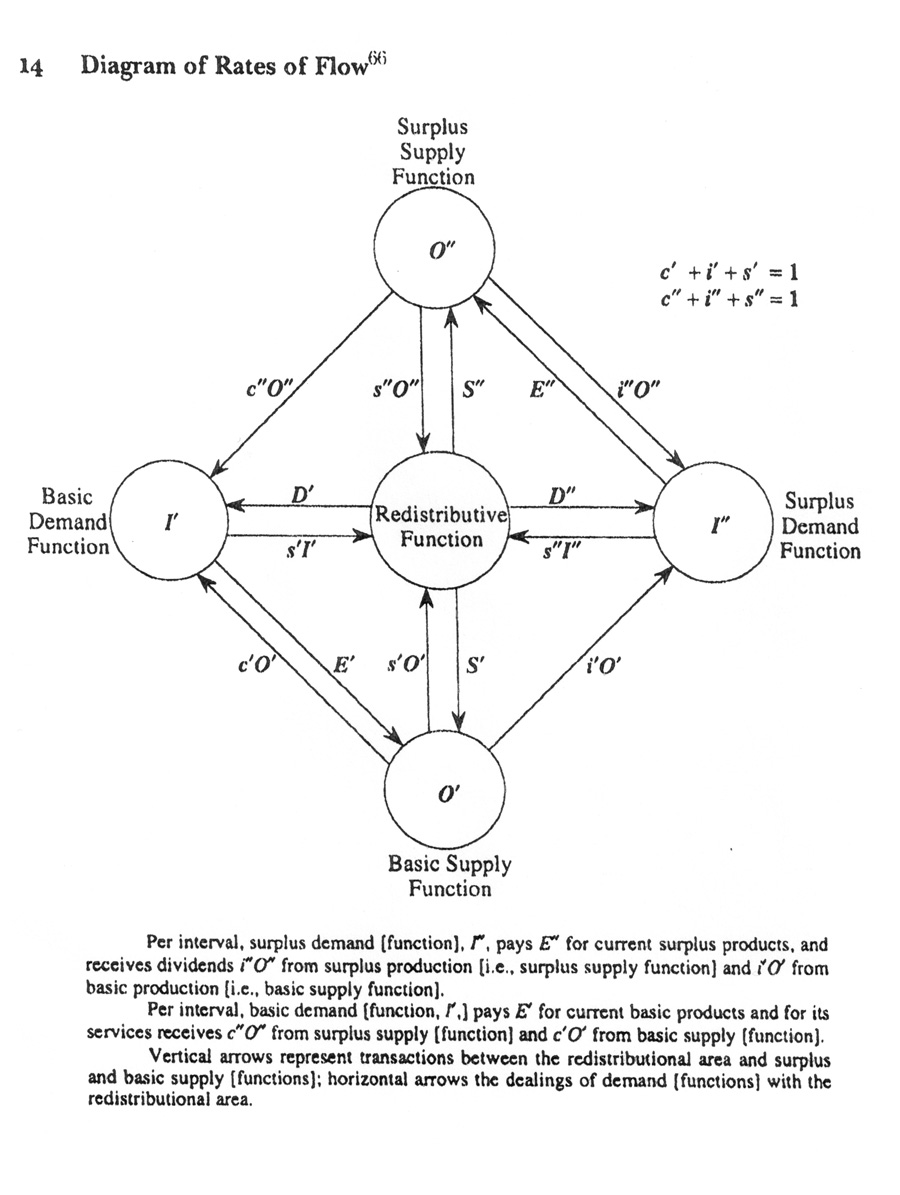# Transfers Between Monetary Functions

(CWL 15, 53-4) This section may be resumed by explaining the diagram of transfers between monetary functions.  In figure 14.1 the reader will notice five circles representing the monetary functions: RD is the redistributive, O’ basic supply, O” surplus supply, I’ basic demand, and I” surplus demand.  In a given interval the action from the redistributive function changes (positively or negatively) the quantities of money available in the other four functions by (S’ – s’O’), (D’ – s’I’), (S” – s”O”), (D” – s”I”), respectively.  In the same interval basic supply makes basic initial payments O’, with c’O’ going to basic demand and i’O’ going to surplus demand.  Similarly, surplus supply makes surplus initial payments O”, with c”O” going to basic demand and i”O” going to surplus demand.  The circuit is completed with basic expenditure E’ going to basic supply, and surplus expenditure E” going to surplus supply.  The other flows in the analysis are given by the equations beneath the diagram:• R’ = E’     (CWL 15, 54)
• R” = E”      (CWL 15, 54)
• I’ = O’ +M’      (CWL 15, 54)
• I” = O” +M”     (CWL 15, 54)
• G = c”O” –i’O’   (CWL 15, 54)
• G = c”O” –i’O’ = 0     the condition of dynamic equilibrium   (CWL 16, 50)
• M’ = (S’ – s’O’) + (D’ – s’I’) + G   (CWL 15, 54)
• M” = (S” – s”O”) + (D” – s”I”) – G   (CWL 15, 54)
• (S’-s’O’) = ΔT’ + (O’ – R’) + ΔR’   (CWL 16, 67)
• (S”- s”O”) = ΔT” + (O” – R”) + ΔR”   (CWL 16, 67)

Thus for example, assuming G = 0, and by the principle of concomitance and by the functional role of credit to bridge time gaps, we have for the basic circuit the relations of functional flows among themselves:

• R’ = E’ = I’ = O’ +M’
• R’ = E’ = I’ = O’ +[S’ – s’O’] + [D’ – s’I’]
• R’ = E’ = I’ = O’ + [ΔT’ + (O’ – R’) + ΔR’] + [D’ – s’I’]

One can develop parallel equations for the surplus circuit, and then combine both circuits to calculate Gross Domestic Functional Flows, GDFF, to replace Gross domestic Product, GDP, as the primary reference for management of the economy. (CWL 15, 53-4)